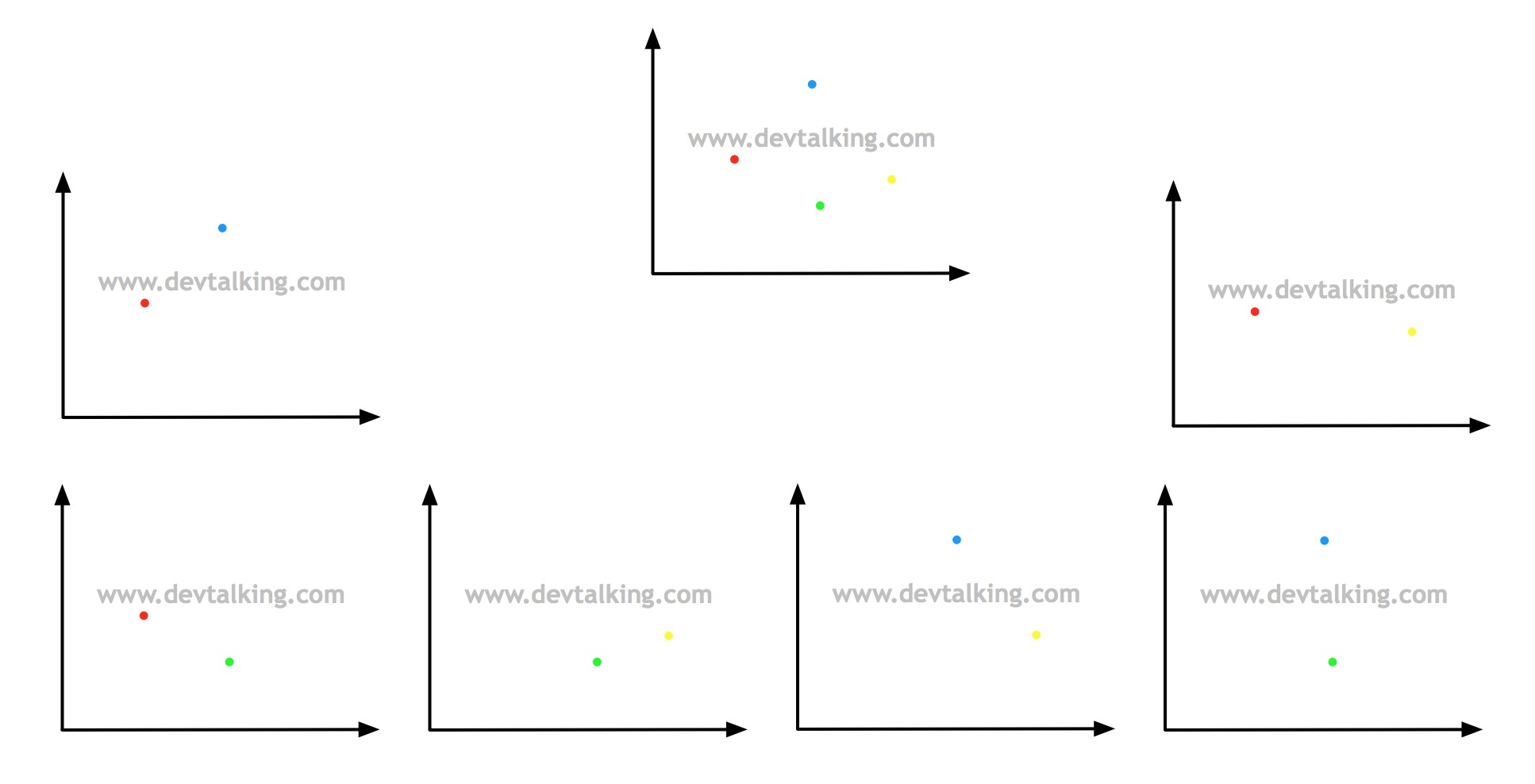决策边界

线性决策边界

$$\hat p = \sigma(\theta^T X_b)=\frac 1 {1+e^{-\theta^{T}X_b}}$$

\hat y =\left\{ \begin{aligned} 1, \ \ \ \hat p \ge 0.5 \\ 0, \ \ \ \hat p < 0.5 \\ \end{aligned} \right.

\hat y =\left\{ \begin{aligned} 1, \ \ \ \ \hat p \ge 0.5, \ \ \ \ \theta^T X_b \ge 0 \\ 0, \ \ \ \ \hat p < 0.5, \ \ \ \ \theta^T X_b < 0 \\ \end{aligned} \right.

$$\theta_0 + \theta_1 X_1 + \theta_2 X_2=0$$

$\theta_0$就是截距，$\theta_1$和$\theta_2$是系数，这个公式绘制出来的是一条直线，这条直线就是能将鸢尾花数据的前两个分类区分开的直线，既线性决策边界。为了能方便的将这条直线绘制出来，我们对上面的公式做一下转换：

$$X_2 = \frac {-\theta_0 - \theta_1 X_1} {\theta_2}$$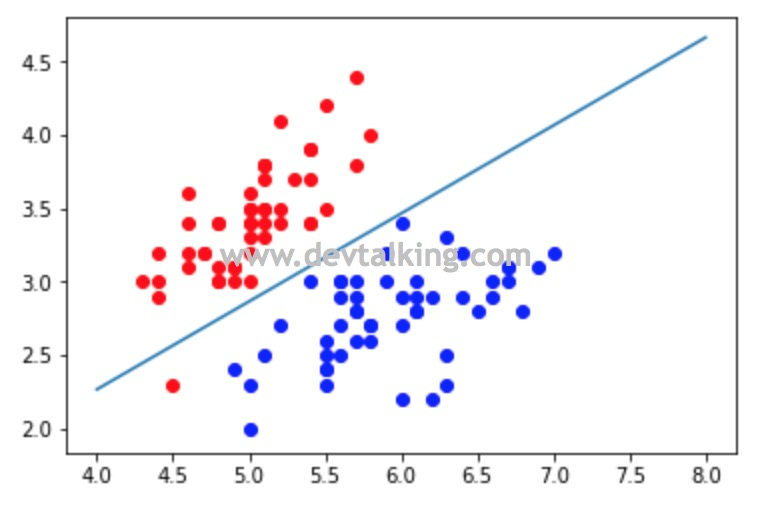不规则决策边界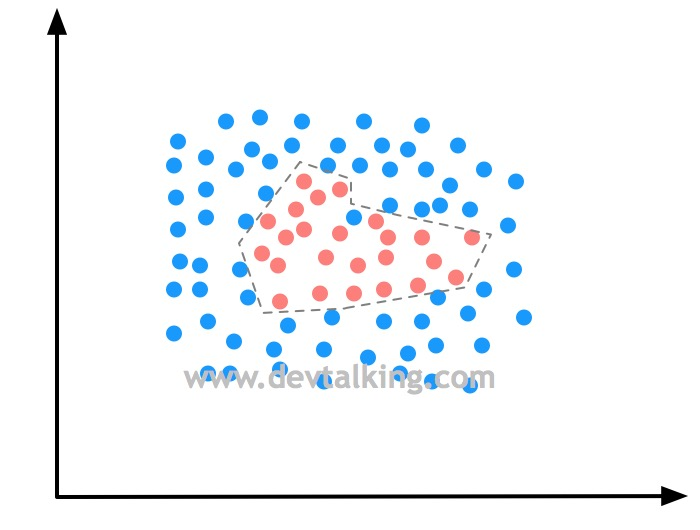• np.meshgrid()这个函数的作用是用给定坐标轴上的点在平面上画格，返回组成网格点的坐标矩阵。
• ravel()方法将高维数组降为一维数组。
• c_[]将两个数组以列的形式拼接起来，形成矩阵。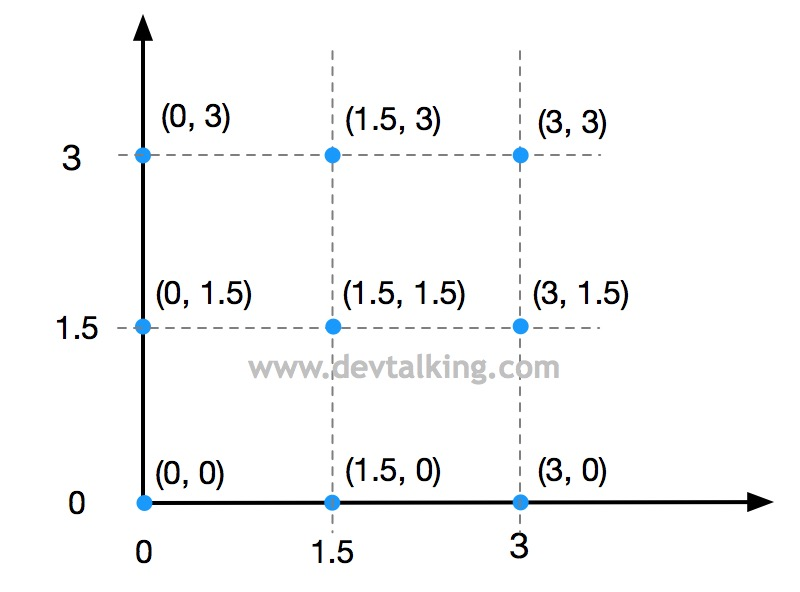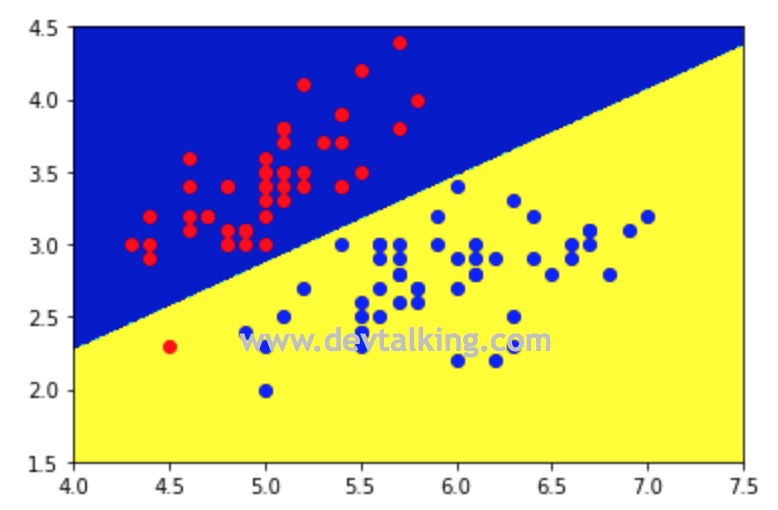kNN的决策边界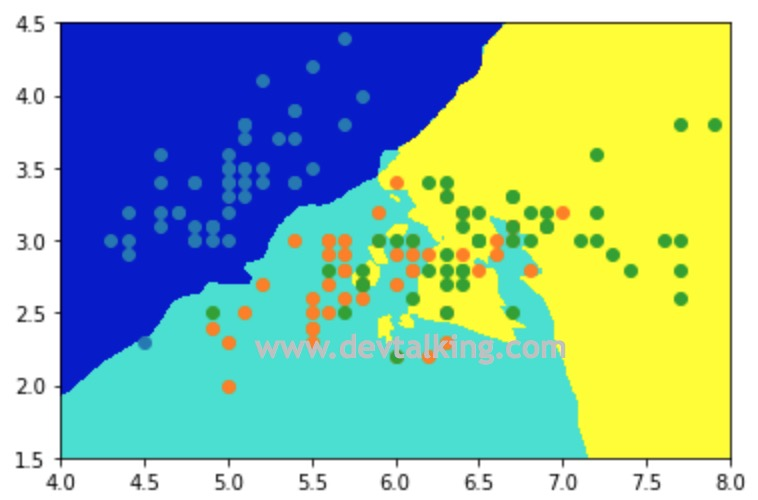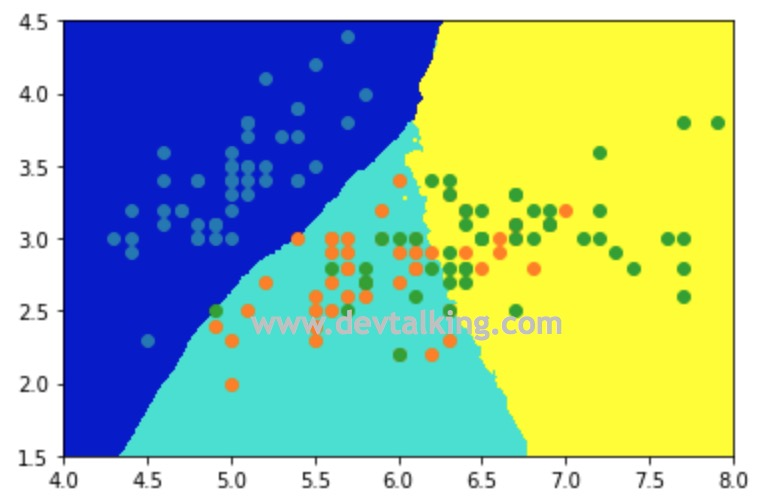逻辑回归中使用多项式特征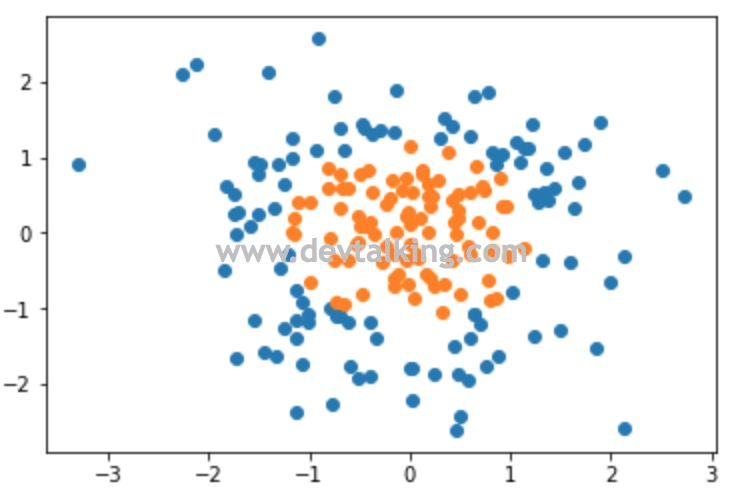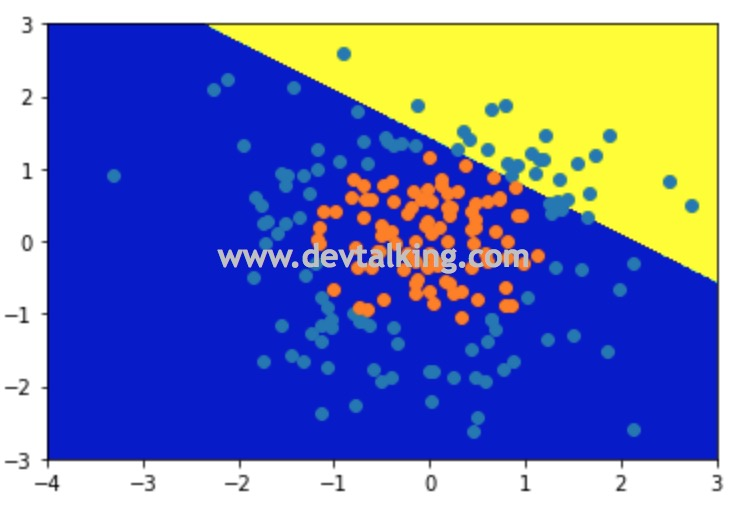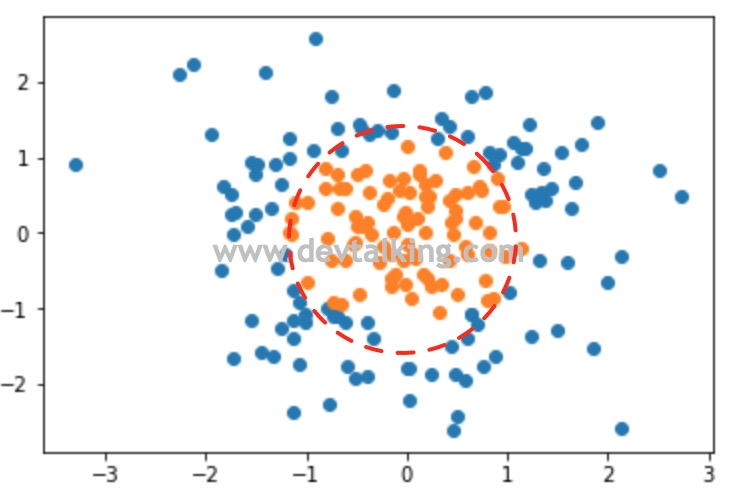$$(x-a)^2 + (y-b)^2 = r^2$$

$a$和$b$是圆心坐标，$r$是半径。那如果我们将上图的圆看作是一个圆心在$(0, 0)$的圆，那么这个圆形的决策边界公式应该就是：

$$x^2 + y^2 -r^2 = 0$$

$$\theta_1 X_1 + \theta_2 X_2 + \theta_0 = 0$$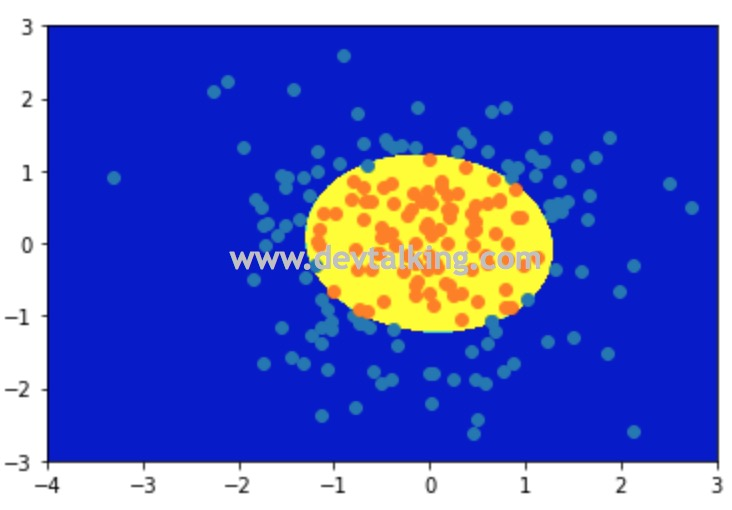逻辑回归中使用模型正则化

$$L(\theta)_{new} = L(\theta) + \alpha L_p$$

$L_p$范数请参见机器学习笔记九之交叉验证、模型正则化

$$L(\theta)_{new} = CL(\theta) + L_p$$

Scikit Learn中的逻辑回归就自带这种形式的模型正则化，下面我们来看一下：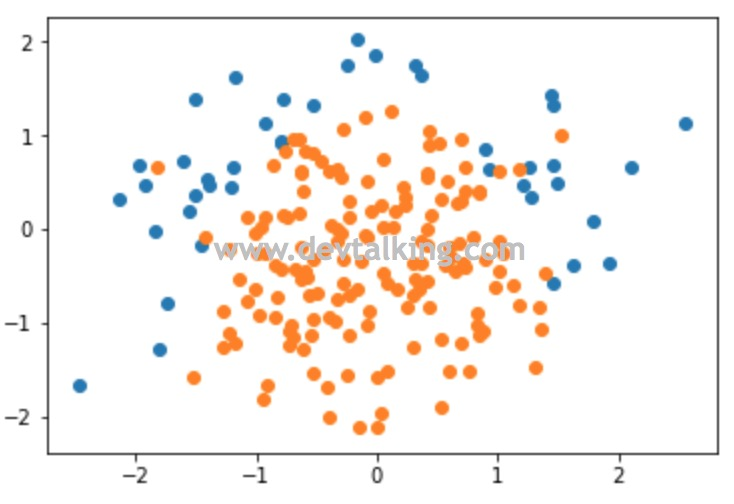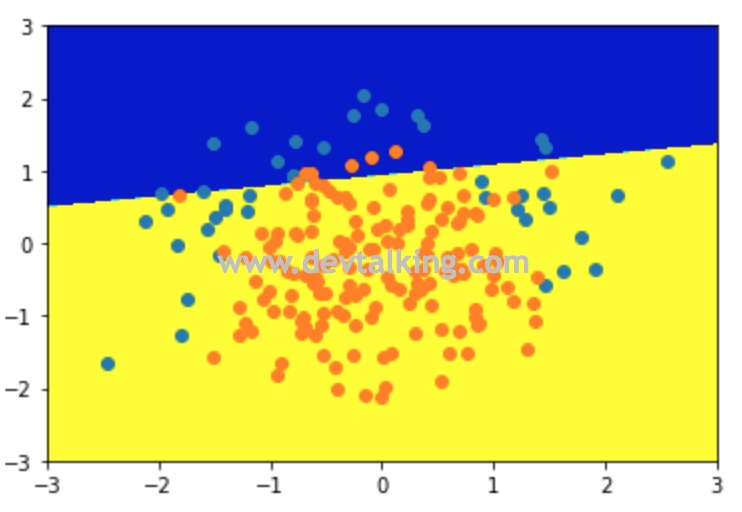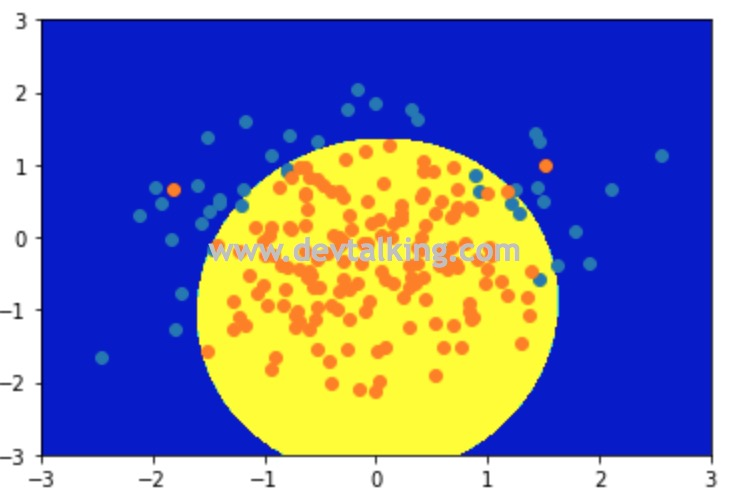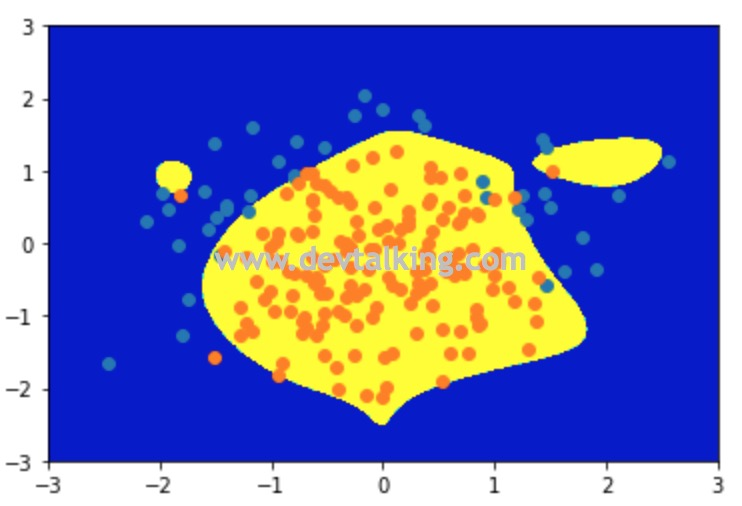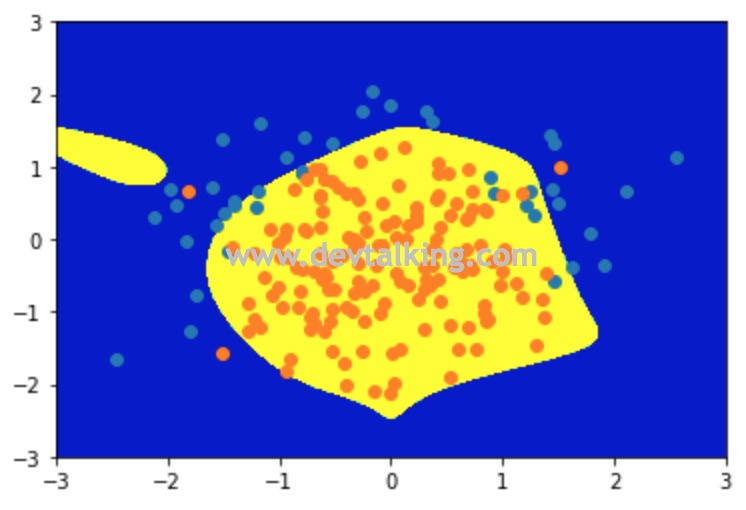逻辑回归解决多分类问题

OvR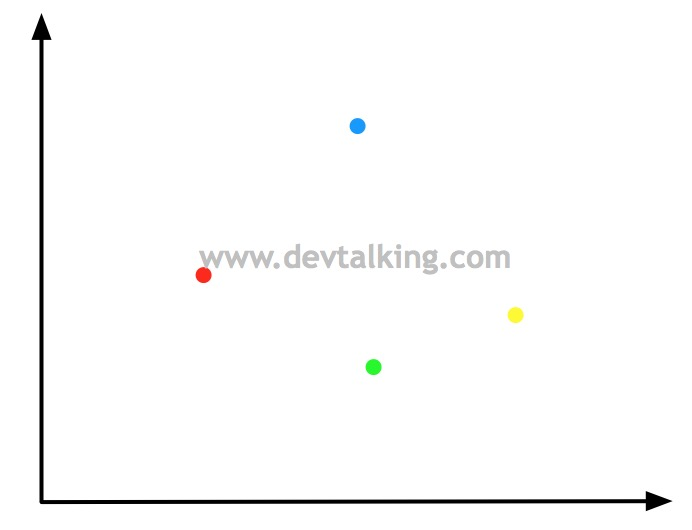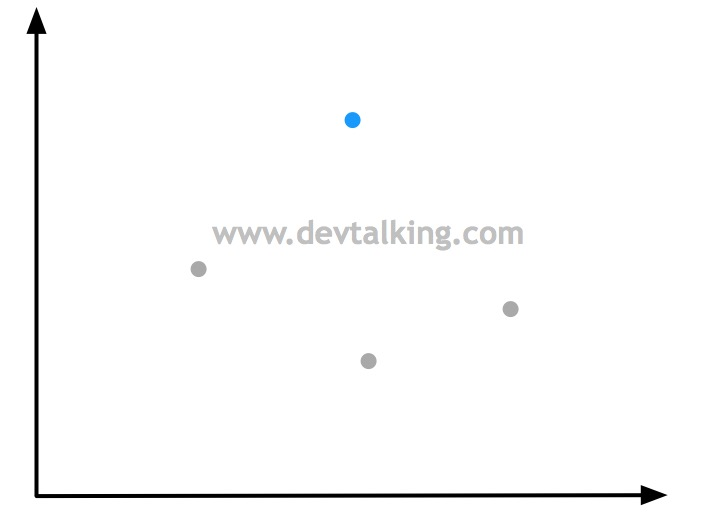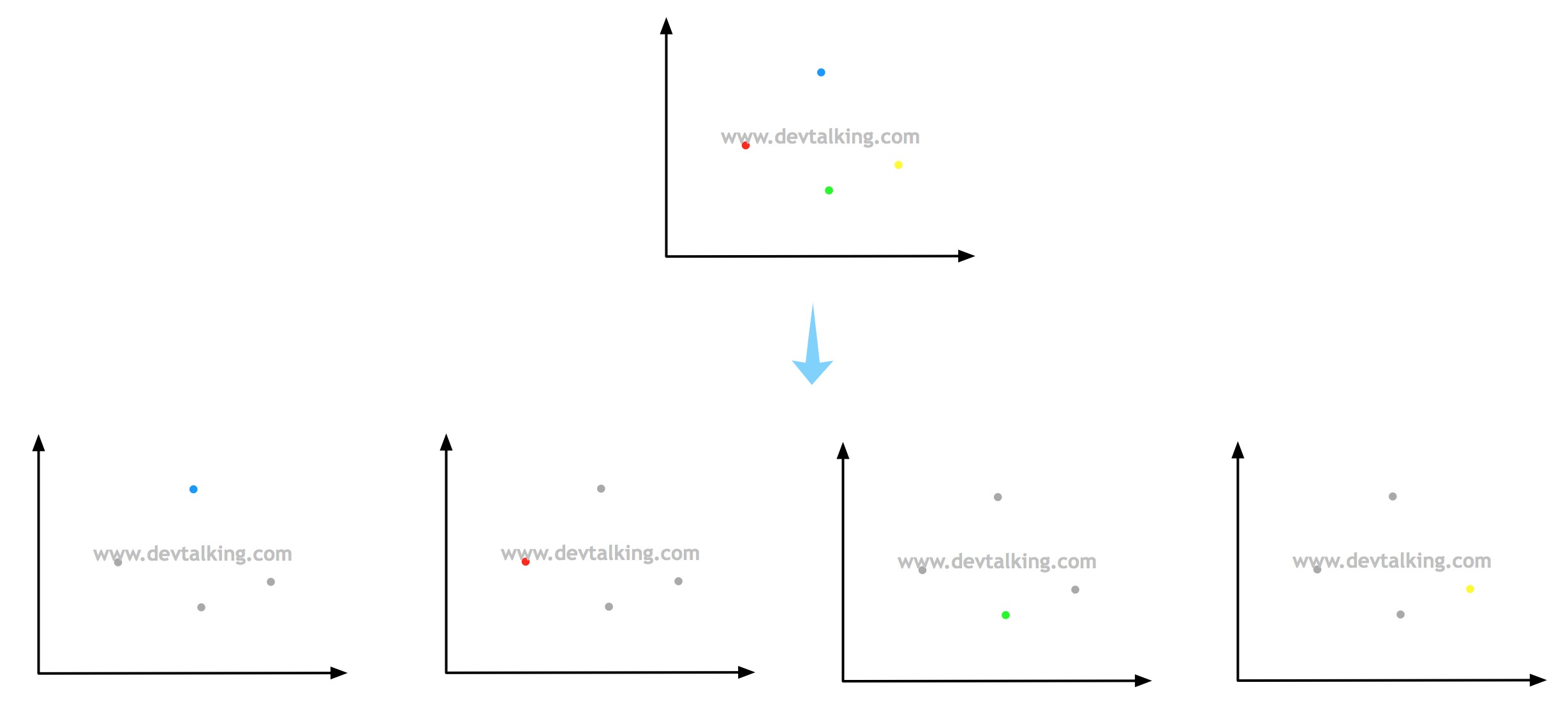OvO